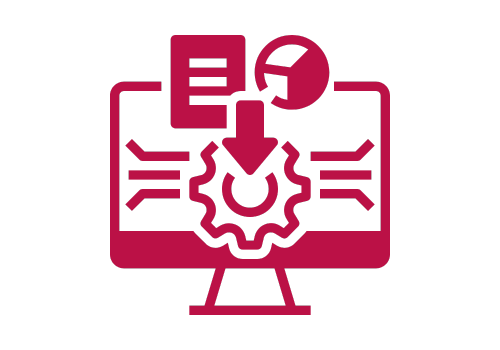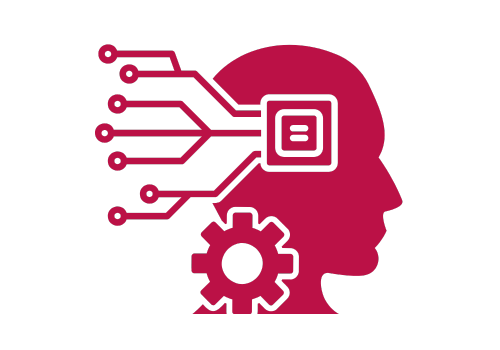## Deep Learning with TensorFlow

Unlock the Power of Deep Learning with Google's Premier Framework
Why This Training?
In an age where machine learning is driving innovation, TensorFlow stands out as the leading framework for building powerful and scalable deep learning models. Whether you're seeking to advance your career, innovate in your company, or dive into the future of computational technologies, understanding TensorFlow is invaluable. Our expert-led training demystifies TensorFlow, guiding you from the basics to advanced functionalities.
Duration: 9 Hours (online / virtual live session)### Who Should Attend?

ML enthusiasts looking to delve into deep learning.
Software developers aiming to integrate machine learning capabilities into applications.
See more
Data scientists wishing to upgrade their model-building toolkit.
Anyone passionate about deep learning and its transformative power.### Course Highlights

Comprehensive overview of TensorFlow and Deep Learning.
Hands-on sessions on building Neural Networks, CNNs, and RNNs.
Dive into TensorFlow with Keras for streamlined model development.
See more
Master data handling, regularization techniques, and model evaluation in TensorFlow.
Insights into Transfer Learning and leveraging TensorFlow Hub.
Guidance on model deployment using TensorFlow Serving.### Pre-requisites:

Basic understanding of Python programming.
Familiarity with fundamental concepts of machine learning (not mandatory, but helpful).

## Materials Required by Participants

A laptop or computer with at least 8GB RAM.
Stable internet connection for virtual sessions (if applicable).
Latest version of TensorFlow installed (installation guidance will be provided before training).
A Python environment (preferably Anaconda) with Jupyter Notebook installed.
Course materials and datasets, which will be shared before the training sessions.

## Introduction to TensorFlow

Objective: Equip participants with foundational knowledge of TensorFlow, its architecture, and applications in building machine learning models.

### 1.TensorFlow Fundamentals (3 hours)

Objective: Understand the basics and significance of TensorFlow in the deep learning ecosystem.
1.1. Introduction to Deep Learning & TensorFlow:
• What is Deep Learning?
• Why TensorFlow? Its place in the ML/DL framework landscape.
• Overview of TensorFlow's ecosystem and components.
1.2. TensorFlow Core Concepts:
• Understanding Tensors: Rank, Shape, and Type.
• Computational Graphs and Sessions.
• Basic operations with Tensors.

1.3. Installing TensorFlow:
• Prerequisites & Setup.
• Validating the installation with a basic program.

1.4. Building a Simple Neural Network with TensorFlow:
• Understanding neural networks: Neurons, Layers, and Activation Functions.
• Implementing a basic neural network for a simple regression problem.

### 2.Diving Deeper into TensorFlow (3 hours)

Objective: Dive into the intricacies of TensorFlow and model development.
2.1. Keras & TensorFlow:
• Introduction to Keras.
• Benefits of using Keras with TensorFlow.
• Building models using the Keras Sequential API.
2.2. Training and Evaluating Models:
• Understanding loss functions and optimizers.
• Evaluating model accuracy and loss.
2.3. Data Handling with TensorFlow:
• Image data handling with tf.data.

2.4. Regularization & Overfitting:
• The challenge of overfitting in deep learning.
• Techniques like Dropout and Batch Normalization.

### 3.Advanced TensorFlow and Applications (3 hours)

Objective: Explore advanced TensorFlow functionalities and real-world applications.
3.1. Convolutional Neural Networks (CNNs) with TensorFlow:
• Understanding CNNs: Filters, Pooling, and Flattening.
• Implementing a basic CNN for image classification.
3.2. Recurrent Neural Networks (RNNs) and LSTM:
• Basics of RNNs and their applications.
• Long Short-Term Memory (LSTM) and its significance.
3.3. TensorFlow Hub & Transfer Learning:
• Leveraging pre-trained models.